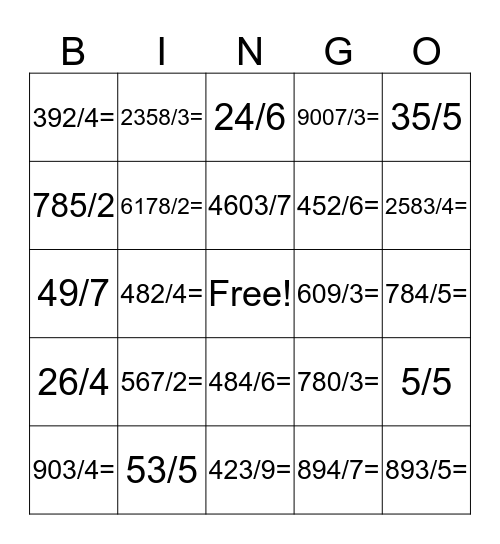# DivisionThis bingo card has a free space and 24 words: 567/2=, 53/5, 4603/7, 903/4=, 35/5, 392/4=, 785/2, 2358/3=, 609/3=, 484/6=, 452/6=, 49/7, 780/3=, 5/5, 6178/2=, 894/7=, 784/5=, 24/6, 2583/4=, 482/4=, 893/5=, 26/4, 9007/3= and 423/9=.

## Play Online